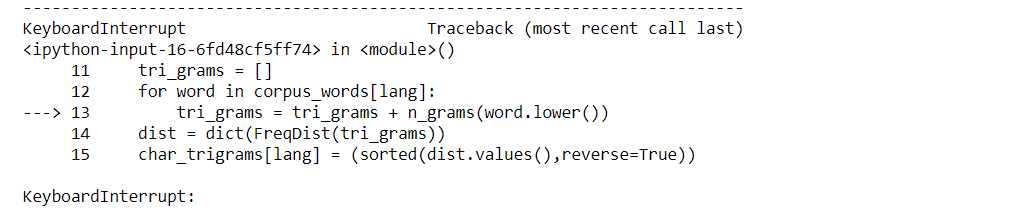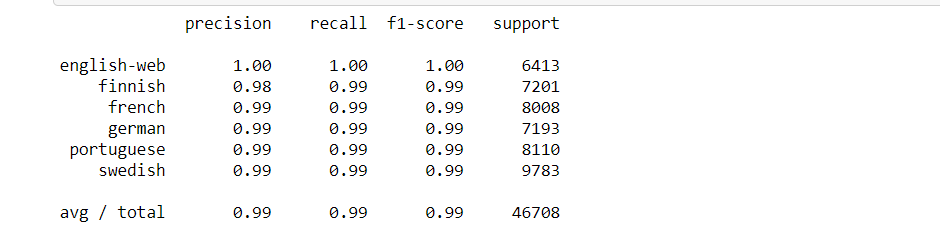Shivani Sharma — August 5, 2021

This article was published as a part of the Data Science Blogathon

## Introduction

The goal of this article is to identify the language from the written text. The text in documents is available in many languages and when we don’t know the language it becomes very difficult sometimes to tell this to google translator as well. For most translators, we have to tell both the input language and the desired language. If you had a text written in Spanish and you only know English or Hindi, how do you identify that the given text is in the Spanish language? So the aim of this; project is to allow users to identify six different languages using NLP(Natural Language Processing). In this article, we perform a comparative analysis between two different approaches concerning accuracy. This is one of the famous projects that every Data Science enthusiast needs to include in their resume.SOURCE

The dataset used for this purpose is the Genesis dataset. Genesis is the famous dataset of the NLTK library. A combination of six languages Finnish, English, German, French, Swedish, and Portuguese is included in this dataset.

```from nltk.corpus import genesis as dataset
languages = ["finnish", "german", "portuguese","english", "french", "swedish"]```

## Approach 1: Identification of language using most popular char-n-grams

In , the authors used the most popular char-n-grams for language detection.

Hypothesis 1: Certain char-n-grams are more frequent in a language than most other char-n-grams.

Hypothesis 1 Validation: Created char-n-gram (only trigrams) to see if there Zipf’s is followed by char-n-grams

```#corpus_words was declared earlier in the notebook - contains words of the 6 languages being considered.
def n_grams(s, n=3):
""""Returns char-n-grams of a words
"""
s = "#"+ s + "#"
return [s[i:i+n] for i in range(len(s)-n+1)]
char_trigrams = {}
for lang in corpus_words.keys():
tri_grams = []
for word in corpus_words[lang]:
tri_grams = tri_grams + n_grams(word.lower())
dist = dict(FreqDist(tri_grams))
char_trigrams[lang] = (sorted(dist.values(),reverse=True))
data = []
for lang in char_trigrams.keys():
data.append(Scatter(
x = range(1, len(char_trigrams[lang])+1),
y = char_trigrams[lang],
name = lang))
iplot({ 'data' : data,
'layout': Layout(title = "Char-tri-gram Frequency Distribution")
})```Hypothesis 1 is validated. There are certain n-grams (tri-grams) that are more frequent than most other char-n-grams.

Method: Divide the data into train-test division (80%-20%). From the training set, most frequent top-k character n-grams. For each document (word-trigrams) in the test set, extract the char-tri-grams and select the language with which there is maximum overlap.

```from random import shuffle
from sklearn.cross_validation import train_test_split
shuffle(tri_gram_dataset)
train_set, test_set = train_test_split(tri_gram_dataset, test_size = 0.20)
print len(train_set), len(test_set)
def get_char_ngram(trigram,k=3):
tri_grams = []
for word in trigram:
tri_grams = tri_grams + n_grams(word.lower())
return tri_grams```
```def top_k_ngrams_features(n=3, k=50):
"""Input: n of the char-n-grams;
k of top-k
Processes Word-Corpus{language: } defined above
Returns the top-k character-n-grams of each language in the form of
{language, }
"""
char_trigrams = {}
for i in train_set:
if i in char_trigrams:
char_trigrams[i] += get_char_ngram(i)
else:
char_trigrams[i] = get_char_ngram(i)
for lang in char_trigrams.keys():
dist = dict(FreqDist(char_trigrams[lang]))
top_k_char_n_gram = (sorted(dist, dist.get, reverse=True))[:100]
char_trigrams[lang] = set(top_k_char_n_gram)
return char_trigrams
char_trigrams = top_k_ngrams_features()
def predict_language_char_ngrams(trigram):
language, max_score = None, -0.1
char_ngrams = get_char_ngram(trigram)
for lang in languages:
if lang == 'english':
lang = 'english-web'
score = float(len(char_trigrams[lang].intersection(char_ngrams)))/float(len(char_ngrams))
if score > max_score:
language = lang
max_score = score
return language```
```y_actual, y_pred = [], []
for i in test_set:
y_actual.append(i)
y_pred.append(predict_language_char_ngrams(i))
print classification_report(y_actual, y_pred)
#Checking Scores for Stop-Words Approach only on TestSet
y_actual, y_pred = test_stopwords_approach(test_set)
print classification_report(y_actual, y_pred)```

#### Observations:

1. There is a certain increase in precision, however, the recall has increased by only 0.02.

2. Approach 2 (Character n-gram) approach might still work better if more n-grams and more data are taken.

## Approach 2: Using Distributed Character-Level Word Representation

### Background:

#### Skip-Gram Model:

In , the authors proposed two neural network models for distributed representation of words – CBOW (Continuous Bag-of-Words Model) and Skip-Gram.

• For the CBOW model, the model takes input ‘n’ before-and-after words and predicts the middle word.

• For the Skip-Gram model, the model takes input a word and predicts ‘n’ before-and-after words of that word.

In the latest work called FastText [3, 4], the CBOW and Skip-Gram models have extended to incorporate character-level information to better understand the text. The major contribution of the work was to modified a word vector to be a sum of the vector of its character n-grams.

```import fasttext
def create_train_file(doc_set,fname):
""" Creates a text file such that for each tri-gram:
where the label is the language. FastText takes a file as input for training.
Returns: The filename of the created file.
"""
train_file = open(fname,"w+")
for i in doc_set:
label = "__label__"+i
text = " ".join(i)
train_file.write(label.encode('utf8')+ " " +text.encode('utf8')+"n")
train_file.close()
return fname
train_filename = create_train_file(train_set,"Train_File.txt")
model = fasttext.supervised(train_filename, 'model',min_count=1,epoch=10,ws=3,
label_prefix='__label__',dim=50)
#For sanity checks
print model.labels
def get_test_pred(test_set):
"""
Input: TestSet :  Pairs
Ouput: List of
"""
y_actual, y_pred = [], []
for i in test_set:
y_actual.append(i)
pred = model.predict([" ".join(i)])
y_pred.append(pred)
return [y_actual, y_pred]```
```y_actual, y_pred = get_test_pred(test_set)
print classification_report(y_actual, y_pred)```

##Observations:

The results of word embedding-based classification are very impressed with the small data like tweets, chats, and short messages.

Note: the rationale for not employing original Skip-gram and CBOW models for language identification is that the limitations of the first models to handle un-seen words were addressed within the FastText paper.

## Conclusions:

1. The stop-word approach is strong and doesn’t require any training but fails when involves short texts.

2. The Char-n-gram approach showed some improvement in short-text datasets but the results weren’t impressive.

3. Word embedding based on the Char-gram can identify the short -texts of a particular language successfully.

4. Hence, for long texts, the stop words approach might be the simplest fit except for short texts, a pre-trained model supported char-n-grams might be used for better results.

This project identifies the language of the text easily and you can also check the comparative analysis of both the approaches 1.Using char-n-grams and 2. Using distributed character-level word representation. Both approaches have their own pros and cons. Prefer the one that is more suitable for you.

This project helps you to enhance your knowledge in NLP and makes your resume stand out. For any queries feel free to

Ping the comment box. You can also reach me out on Linkedin-https://www.linkedin.com/in/shivani-sharma-aba6141b6/

Stay tuned to AnalyticsVidya for my upcoming articles.